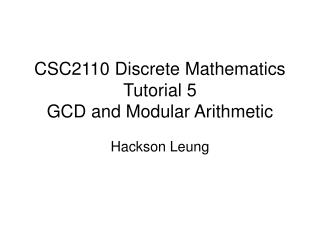DownloadDownload PresentationCSC2110 Discrete Mathematics Tutorial 5 GCD and Modular Arithmetic

# CSC2110 Discrete Mathematics Tutorial 5 GCD and Modular Arithmetic

Download Presentation## CSC2110 Discrete Mathematics Tutorial 5 GCD and Modular Arithmetic

- - - - - - - - - - - - - - - - - - - - - - - - - - - E N D - - - - - - - - - - - - - - - - - - - - - - - - - - -
##### Presentation Transcript

1. CSC2110 Discrete MathematicsTutorial 5GCD and Modular Arithmetic Hackson Leung

2. Agenda • Greatest Common Divisor • Euclid’s Algorithm • Extended Euclid’s Algorithm • Modular Arithmetic • Basic Manipulations • Multiplicative Inverse • Fermat’s Little Theorem • Wilson’s Theorem

3. Number Theory • Throughout the whole tutorial, we assume, unless otherwise specified, that all variables are integers

4. Euclid’s Algorithm • Main idea: • So we iteratively do divisions • And is gcd of and

5. Euclid’s Algorithm • Example 1 • Find gcd(2110, 1130)

6. Euclid’s Algorithm • Example 2 • Given two sticks • By elongating the sticks with same length, find the smallest positive difference in length between the two stick piles Length = 2020 Length = 2100

7. Euclid’s Algorithm • Example 2 • Observation: We want to minimize positive z such that • Hint: spc(a, b) = gcd(a, b) • Extension 1: If we allow z to be non-negative, • Can z be even smaller? • Shortest length of stick piles, respectively?

8. Extended Euclid’s Algorithm • Example 2 (Extension 2) • I want to know how many sticks of each of two lengths so that z > 0 is minimized • Things on hand: • Want to know:

9. Extended Euclid’s Algorithm • Key: Trace from the steps of Euclid’s algorithm • gcd(2100, 2020) = 20

10. Extended Euclid’s Algorithm • Key: Trace from the steps of Euclid’s algorithm

11. Modular Arithmetic • Know what it means, first! • Which means • Which means • a and b have same remainder when divided by n

12. Basic Manipulations • Given

13. Basic Manipulations • Examples

14. Basic Manipulations • Example • Using modular arithmetic, prove that a positive integer N is divisible by 3 if and only if sum of digits is divisible by 3

15. Basic Manipulations • We can express N in the following way • We can say • Since , hence • Conclusion:

16. Multiplicative Inverse • Definition: • We say A’ is the multiplicative inverse of A modulo N • Theorem: • A’ exists if and only if • We also say that A and N are co-prime • Note: N is NOT necessarily prime

17. Multiplicative Inverse • Example • Find the multiplicative inverse of 211 modulo 101

18. Fermat’s Little Theorem • If p is prime and a is not multiple of p, then • Example 1: Calculate • Are 2110 and 1009 co-prime? • If so, by the theorem, • By multiplication rule, • Same as finding • Ans:

19. Fermat’s Little Theorem • Example 2 • Show that, if p is prime and co-prime with a, the multiplicative inverse of a modulo p, denoted by , has the same remainder as when divided by p. • Observation • By the theorem and multiplication rule, we can say

20. Fermat’s Little Theorem • Example 2 (Cont’d) • Observation • By the theorem and multiplication rule, we can say • Then,

21. Wilson’s Theorem • It states that • What if p is not prime? • p = 4, trivial • p > 5,

22. Wilson’s Theorem • What if p is prime? • Remember the proof of Fermat’s Little Theorem? • shows a permutation of • Write them down in the yth column of a table • Each row and column has exactly a single 1 • Pair up and it becomes • Only for y = 1 and y = p-1, • So,

23. The End